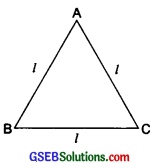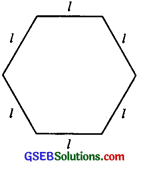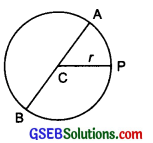# GSEB Solutions Class 6 Maths Chapter 11 Algebra Ex 11.2

Gujarat Board GSEB Textbook Solutions Class 6 Maths Chapter 11 Algebra Ex 11.2 Textbook Questions and Answers.

## Gujarat Board Textbook Solutions Class 6 Maths Chapter 11 Algebra Ex 11.2

Question 1.
The side of an equilateral triangle is shown by l. Express the perimeter of the equilateral triangle using l.Solution:
The side of the equilateral triangle ABC = l
∴ Its perimeter = AB + BC + AC
= l + l + l = 3l
∵ The perimeter of an equilateral triangle = 3l
Note: 3 x l, 3.l, and 3l are the same. They represent the product of 3 and l.Question 2.
The side of a regular hexagon in the figure is denoted by l. Express the perimeter of the hexagon using l.Hint: A regular hexagon has all its six sides equal in length.
Solution:
Since all the sides of a regular hexagon are equal.
∵ Each side of the hexagon = l
∴ Its perimeter = l + l + l + l + l + l = 6lQuestion 3.
A cube is a three-dimensional figure as shown here. It has six faces and all. of them are identical squares. The length of an edge of the cube is given by l. Find the formula for the total length of the edges of a cube.Solution:
A cube has six identical faces. It has 12 edges. Each edge has an equal length.
∴ The total length of edges = 12 × l or 12lQuestion 4.
The diameter of a circle is a line that joins two points on the circle and also passes through the centre of the circle.
(In the adjoining figure, AB is the diameter of the circle; C is its centre.) Express the diameter of the circle (d) in terms of its radius (r).Solution:
Radius = r and diameter = d
Since the diameter of a circle is double the radius.
∴ Diameter = 2 × radius
or d = 2 × r or d = 2rQuestion 5.
To find the sum of three numbers 14, 27, and 13 we can have two ways:
(a) We may first add 14 and 27 to get 41 and then add 13 to it to get the total sum of 54 or
(b) We may add 27 and 13 to get 40 and then add 14 to get the sum of 54. Thus,
(14+27) + 13 = 14 + (27 + 13).
This can be done for any three numbers. This property is known as the associativity of the addition of numbers. Express this property which we have already studied in the chapter on Whole Numbers, in a general way, by using variables a, b and c.
Solution:
Let the three numbers be ‘a’, ‘b’ and ‘c’ Then, the property-‘associativity of addition’ can be expressed as:
a + (b + c) = (a + b) + c